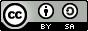# 语义角色标注¶

## 说明¶

1. 本教程可支持在 CPU/GPU 环境下运行

2. Docker镜像支持的CUDA/cuDNN版本

如果使用了Docker运行Book，请注意：这里所提供的默认镜像的GPU环境为 CUDA 8/cuDNN 5，对于NVIDIA Tesla V100等要求CUDA 9的 GPU，使用该镜像可能会运行失败;

3. 文档和脚本中代码的一致性问题

请注意：为使本文更加易读易用，我们拆分、调整了train.py的代码并放入本文。本文中代码与train.py的运行结果一致，可直接运行train.py进行验证。

## 背景介绍¶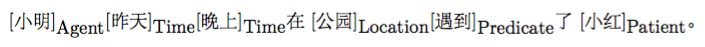1. 构建一棵句法分析树，例如，图1是对上面例子进行依存句法分析得到的一棵句法树。
2. 从句法树上识别出给定谓词的候选论元。
3. 候选论元剪除；一个句子中的候选论元可能很多，候选论元剪除就是从大量的候选项中剪除那些最不可能成为论元的候选项。
4. 论元识别：这个过程是从上一步剪除之后的候选中判断哪些是真正的论元，通常当做一个二分类问题来解决。
5. 对第4步的结果，通过多分类得到论元的语义角色标签。可以看到，句法分析是基础，并且后续步骤常常会构造的一些人工特征，这些特征往往也来自句法分析。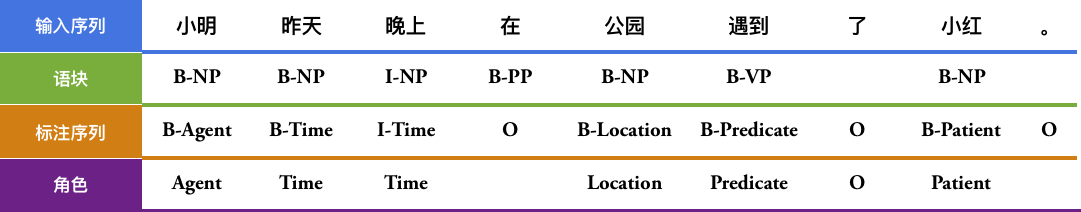## 模型概览¶

### 栈式循环神经网络（Stacked Recurrent Neural Network）¶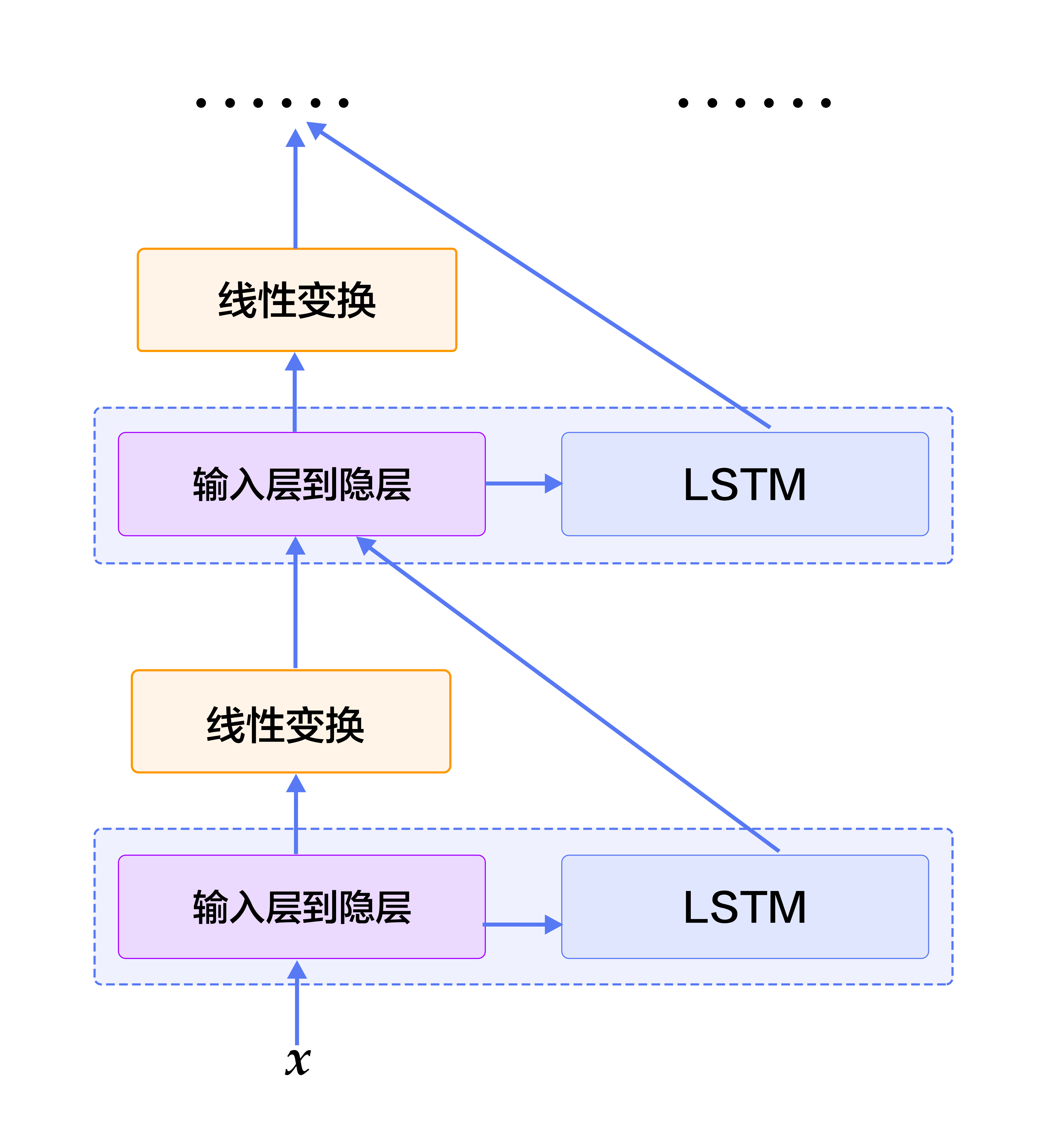### 双向循环神经网络（Bidirectional Recurrent Neural Network）¶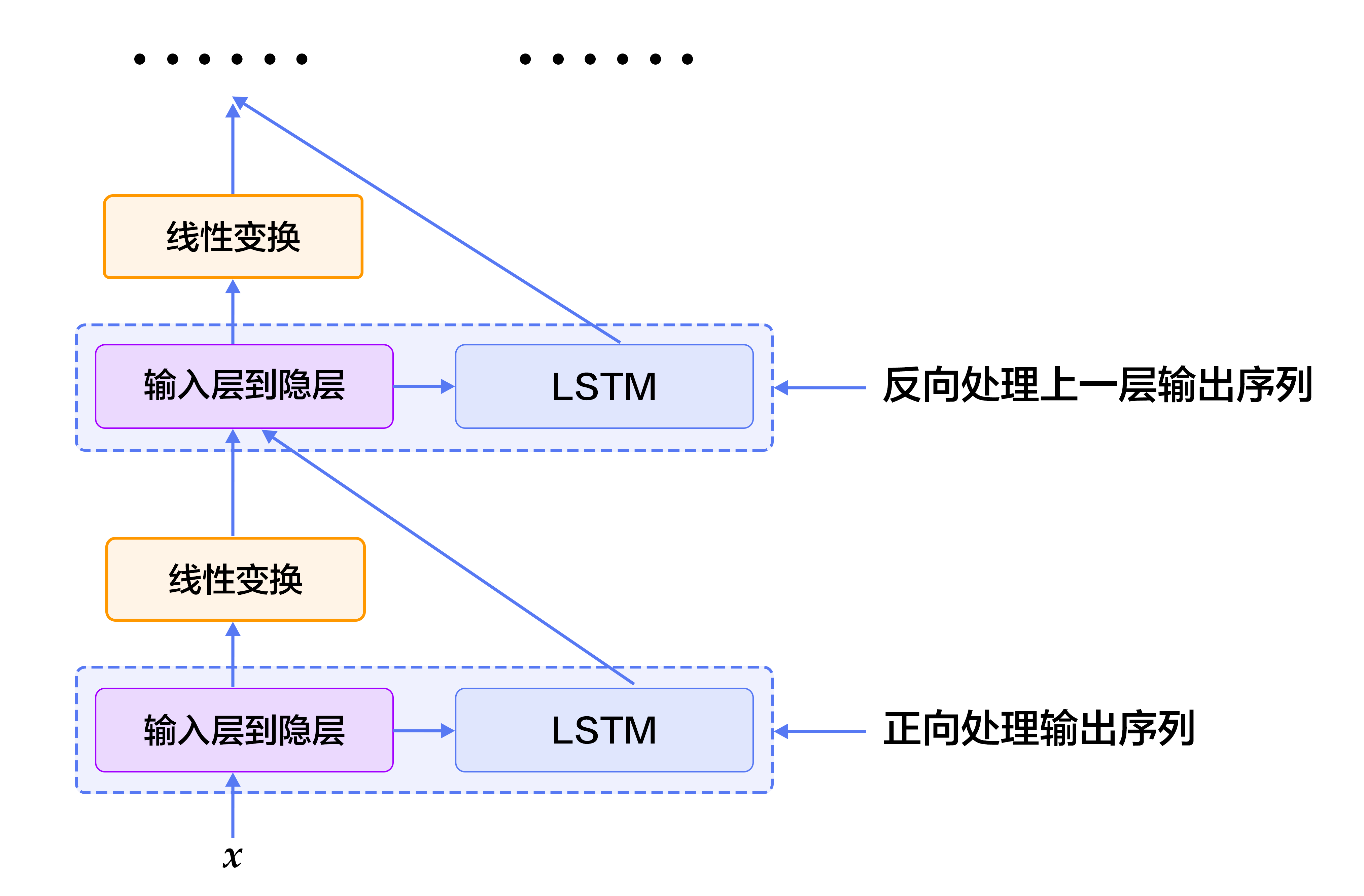### 条件随机场 (Conditional Random Field)¶

CRF是一种概率化结构模型，可以看作是一个概率无向图模型，结点表示随机变量，边表示随机变量之间的概率依赖关系。简单来讲，CRF学习条件概率$P(X|Y)$，其中 $X = (x_1, x_2, ... , x_n)$ 是输入序列，$Y = (y_1, y_2, ... , y_n)$ 是标记序列；解码过程是给定 $X$序列求解令$P(Y|X)$最大的$Y$序列，即$Y^* = \mbox{arg max}_{Y} P(Y | X)$。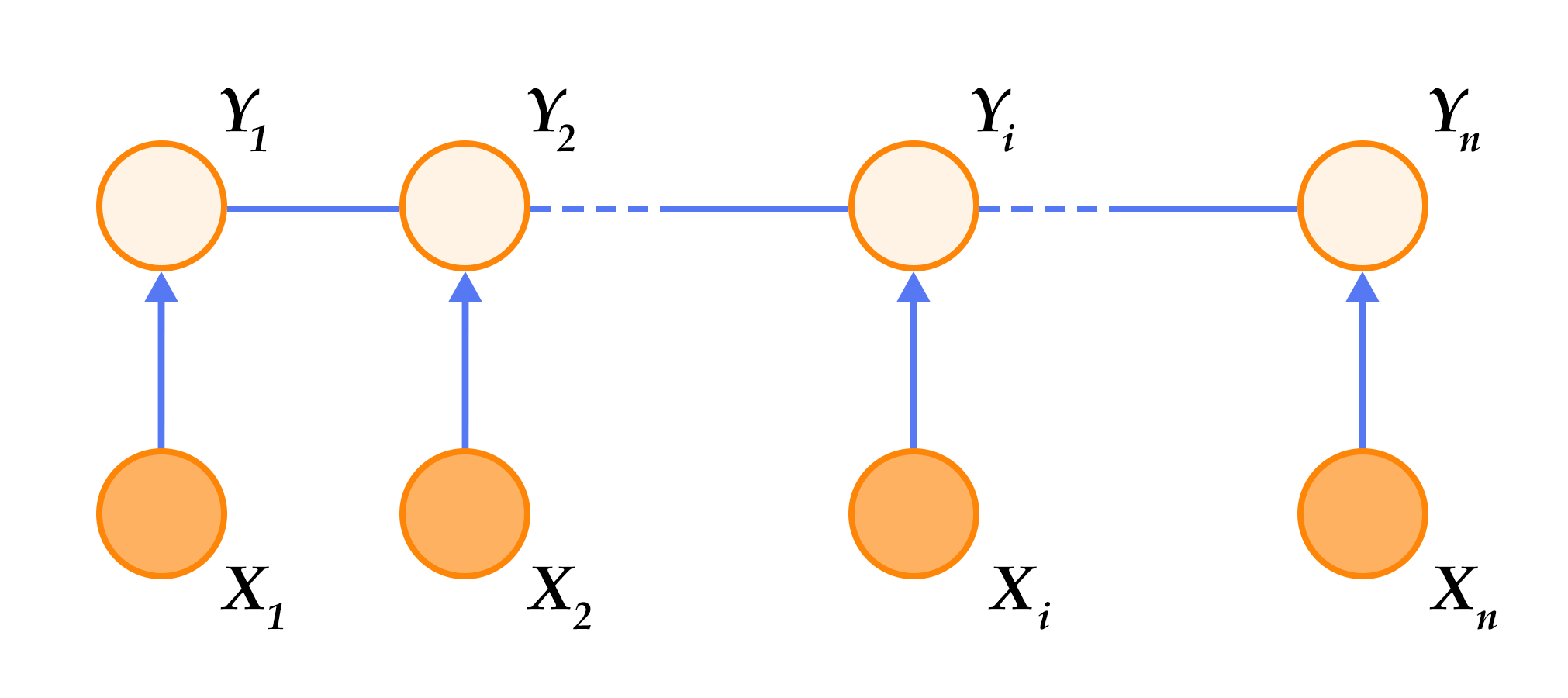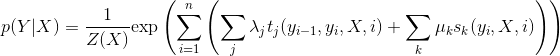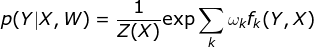$\omega$是特征函数对应的权值，是CRF模型要学习的参数。训练时，对于给定的输入序列和对应的标记序列集合$D = \left[(X_1, Y_1), (X_2 , Y_2) , ... , (X_N, Y_N)\right]$ ，通过正则化的极大似然估计，求解如下优化目标：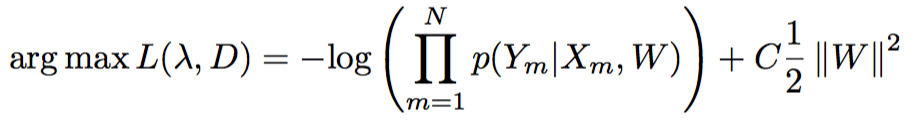### 深度双向LSTM（DB-LSTM）SRL模型¶

1. 构造输入；
• 输入1是谓词，输入2是句子
• 将输入1扩展成和输入2一样长的序列，用one-hot方式表示；
1. one-hot方式的谓词序列和句子序列通过词表，转换为实向量表示的词向量序列；
2. 将步骤2中的2个词向量序列作为双向LSTM的输入，学习输入序列的特征表示；
3. CRF以步骤3中模型学习到的特征为输入，以标记序列为监督信号，实现序列标注；

• 谓词上下文：上面的方法中，只用到了谓词的词向量表达谓词相关的所有信息，这种方法始终是非常弱的，特别是如果谓词在句子中出现多次，有可能引起一定的歧义。从经验出发，谓词前后若干个词的一个小片段，能够提供更丰富的信息，帮助消解歧义。于是，我们把这样的经验也添加到模型中，为每个谓词同时抽取一个“谓词上下文” 片段，也就是从这个谓词前后各取$n$个词构成的一个窗口片段；
• 谓词上下文区域标记：为句子中的每一个词引入一个0-1二值变量，表示它们是否在“谓词上下文”片段中；

1. 构造输入
• 输入1是句子序列，输入2是谓词序列，输入3是谓词上下文，从句子中抽取这个谓词前后各$n$个词，构成谓词上下文，用one-hot方式表示，输入4是谓词上下文区域标记，标记了句子中每一个词是否在谓词上下文中；
• 将输入2~3均扩展为和输入1一样长的序列；
1. 输入1~4均通过词表取词向量转换为实向量表示的词向量序列；其中输入1、3共享同一个词表，输入2和4各自独有词表；
2. 第2步的4个词向量序列作为双向LSTM模型的输入；LSTM模型学习输入序列的特征表示，得到新的特性表示序列；
3. CRF以第3步中LSTM学习到的特征为输入，以标记序列为监督信号，完成序列标注；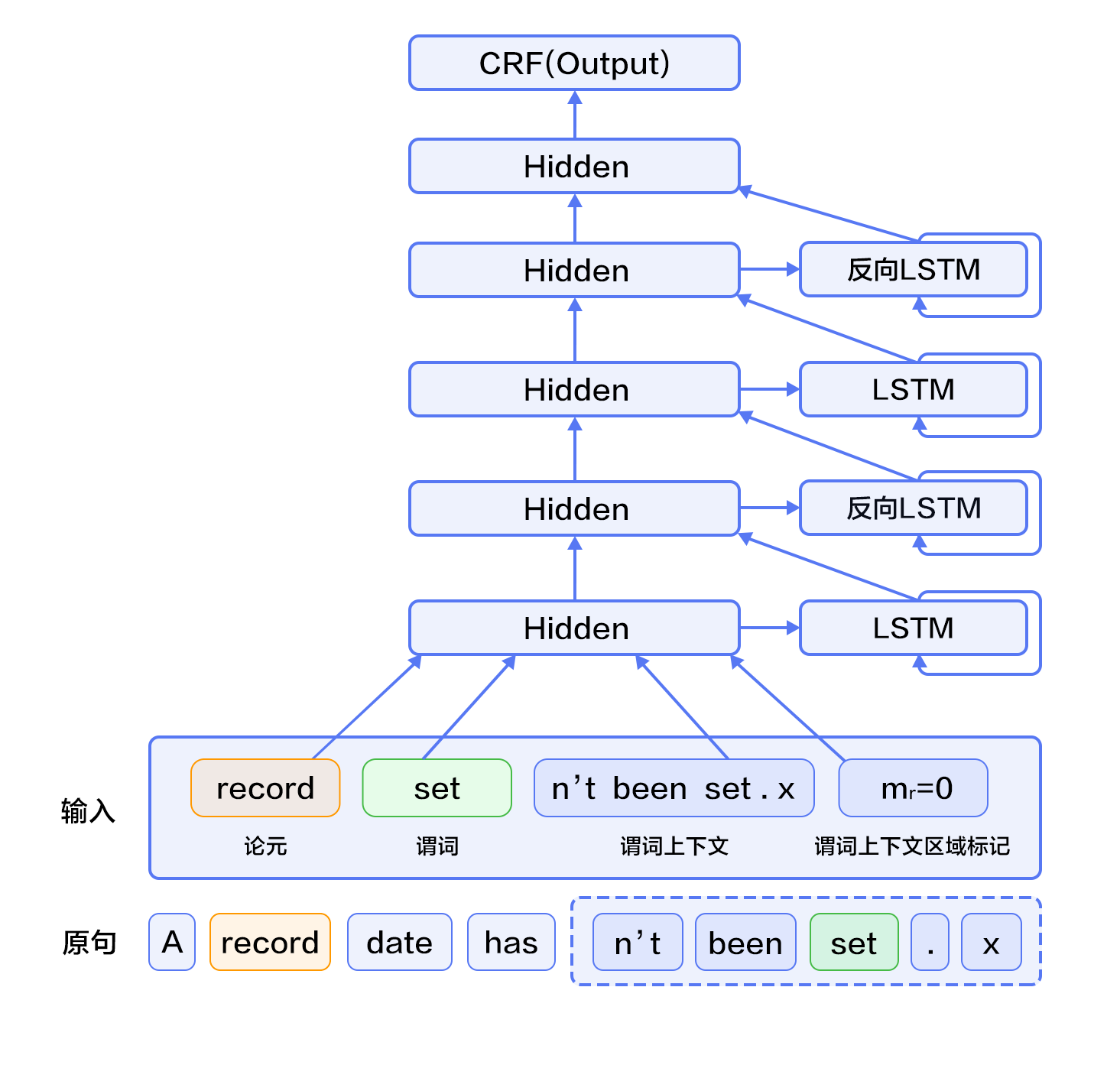## 数据介绍¶

conll05st-release/
└── test.wsj
├── props  # 标注结果
└── words  # 输入文本序列


1. 将文本序列和标记序列其合并到一条记录中；
2. 一个句子如果含有$n$个谓词，这个句子会被处理$n$次，变成$n$条独立的训练样本，每个样本一个不同的谓词；
3. 抽取谓词上下文和构造谓词上下文区域标记；
4. 构造以BIO法表示的标记；
5. 依据词典获取词对应的整数索引。

A set n't been set . × 0 B-A1
record set n't been set . × 0 I-A1
date set n't been set . × 0 I-A1
has set n't been set . × 0 O
n't set n't been set . × 1 B-AM-NEG
been set n't been set . × 1 O
set set n't been set . × 1 B-V
. set n't been set . × 1 O

word_dict 输入句子的词典，共计44068个词
label_dict 标记的词典，共计106个标记
predicate_dict 谓词的词典，共计3162个词
emb 一个训练好的词表，32维

from __future__ import print_function

import math, os
import numpy as np
import six
import time

with_gpu = os.getenv('WITH_GPU', '0') != '0'

word_dict, verb_dict, label_dict = conll05.get_dict()
word_dict_len = len(word_dict)
label_dict_len = len(label_dict)
pred_dict_len = len(verb_dict)

print('word_dict_len: ', word_dict_len)
print('label_dict_len: ', label_dict_len)
print('pred_dict_len: ', pred_dict_len)


## 模型配置说明¶

• 定义输入数据维度及模型超参数。
mark_dict_len = 2    # 谓上下文区域标志的维度，是一个0-1 2值特征，因此维度为2
word_dim = 32        # 词向量维度
mark_dim = 5         # 谓词上下文区域通过词表被映射为一个实向量，这个是相邻的维度
hidden_dim = 512     # LSTM隐层向量的维度 ： 512 / 4
depth = 8            # 栈式LSTM的深度
mix_hidden_lr = 1e-3 # linear_chain_crf层的基础学习率

IS_SPARSE = True     # 是否以稀疏方式更新embedding
PASS_NUM = 10        # 训练轮数
BATCH_SIZE = 10      # batch size 大小

embedding_name = 'emb'


• 如上文提到，我们用基于英文维基百科训练好的词向量来初始化序列输入、谓词上下文总共6个特征的embedding层参数，在训练中不更新。
# 这里加载PaddlePaddle保存的二进制参数
with open(file_name, 'rb') as f:
return np.fromfile(f, dtype=np.float32).reshape(h, w)


## 训练模型¶

• 我们根据网络拓扑结构和模型参数来进行训练，在构造时还需指定优化方法，这里使用最基本的SGD方法(momentum设置为0)，同时设定了学习率、正则等。

use_cuda = False #在cpu上执行训练
save_dirname = "label_semantic_roles.inference.model" #训练得到的模型参数保存在文件中
is_local = True


### 数据输入层定义¶

# 句子序列
word = fluid.data(
name='word_data', shape=[None, 1], dtype='int64', lod_level=1)

# 谓词
predicate = fluid.data(
name='verb_data', shape=[None, 1], dtype='int64', lod_level=1)

# 谓词上下文5个特征
ctx_n2 = fluid.data(
name='ctx_n2_data', shape=[None, 1], dtype='int64', lod_level=1)
ctx_n1 = fluid.data(
name='ctx_n1_data', shape=[None, 1], dtype='int64', lod_level=1)
ctx_0 = fluid.data(
name='ctx_0_data', shape=[None, 1], dtype='int64', lod_level=1)
ctx_p1 = fluid.data(
name='ctx_p1_data', shape=[None, 1], dtype='int64', lod_level=1)
ctx_p2 = fluid.data(
name='ctx_p2_data', shape=[None, 1], dtype='int64', lod_level=1)

# 谓词上下区域标志
mark = fluid.data(
name='mark_data', shape=[None, 1], dtype='int64', lod_level=1)


### 定义网络结构¶

#预训练谓词和谓词上下区域标志
predicate_embedding = fluid.embedding(
input=predicate,
size=[pred_dict_len, word_dim],
dtype='float32',
is_sparse=IS_SPARSE,
param_attr='vemb')

mark_embedding = fluid.embedding(
input=mark,
size=[mark_dict_len, mark_dim],
dtype='float32',
is_sparse=IS_SPARSE)

#句子序列和谓词上下文5个特征并预训练
word_input = [word, ctx_n2, ctx_n1, ctx_0, ctx_p1, ctx_p2]
# 因词向量是预训练好的，这里不再训练embedding表，
# 参数属性trainable设置成False阻止了embedding表在训练过程中被更新
emb_layers = [
fluid.embedding(
size=[word_dict_len, word_dim],
input=x,
param_attr=fluid.ParamAttr(
name=embedding_name, trainable=False)) for x in word_input
]
#加入谓词和谓词上下区域标志的预训练结果
emb_layers.append(predicate_embedding)
emb_layers.append(mark_embedding)


# 共有8个LSTM单元被训练，每个单元的方向为从左到右或从右到左，
# 由参数is_reverse确定
# 第一层栈结构
hidden_0_layers = [
fluid.layers.fc(input=emb, size=hidden_dim, act='tanh')
for emb in emb_layers
]

hidden_0 = fluid.layers.sums(input=hidden_0_layers)

lstm_0 = fluid.layers.dynamic_lstm(
input=hidden_0,
size=hidden_dim,
candidate_activation='relu',
gate_activation='sigmoid',
cell_activation='sigmoid')

# 用直连的边来堆叠L-LSTM、R-LSTM
input_tmp = [hidden_0, lstm_0]

# 其余的栈结构
for i in range(1, depth):
mix_hidden = fluid.layers.sums(input=[
fluid.layers.fc(input=input_tmp, size=hidden_dim, act='tanh'),
fluid.layers.fc(input=input_tmp, size=hidden_dim, act='tanh')
])

lstm = fluid.layers.dynamic_lstm(
input=mix_hidden,
size=hidden_dim,
candidate_activation='relu',
gate_activation='sigmoid',
cell_activation='sigmoid',
is_reverse=((i % 2) == 1))

input_tmp = [mix_hidden, lstm]

# 取最后一个栈式LSTM的输出和这个LSTM单元的输入到隐层映射，
# 经过一个全连接层映射到标记字典的维度，来学习 CRF 的状态特征
feature_out = fluid.layers.sums(input=[
fluid.layers.fc(input=input_tmp, size=label_dict_len, act='tanh'),
fluid.layers.fc(input=input_tmp, size=label_dict_len, act='tanh')
])

# 标注序列
target = fluid.data(
name='target', shape=[None, 1], dtype='int64', lod_level=1)

# 学习 CRF 的转移特征
crf_cost = fluid.layers.linear_chain_crf(
input=feature_out,
label=target,
param_attr=fluid.ParamAttr(
name='crfw', learning_rate=mix_hidden_lr))

avg_cost = fluid.layers.mean(crf_cost)

# 使用最基本的SGD优化方法(momentum设置为0)
sgd_optimizer = fluid.optimizer.SGD(
learning_rate=fluid.layers.exponential_decay(
learning_rate=0.01,
decay_steps=100000,
decay_rate=0.5,
staircase=True))

sgd_optimizer.minimize(avg_cost)


crf_decode = fluid.layers.crf_decoding(
input=feature_out, param_attr=fluid.ParamAttr(name='crfw'))

batch_size=BATCH_SIZE)

place = fluid.CUDAPlace(0) if use_cuda else fluid.CPUPlace()


feeder = fluid.DataFeeder(
feed_list=[
word, ctx_n2, ctx_n1, ctx_0, ctx_p1, ctx_p2, predicate, mark, target
],
place=place)
exe = fluid.Executor(place)


main_program = fluid.default_main_program()

exe.run(fluid.default_startup_program())
embedding_param = fluid.global_scope().find_var(
embedding_name).get_tensor()
embedding_param.set(
place)

start_time = time.time()
batch_id = 0
for pass_id in six.moves.xrange(PASS_NUM):
for data in train_data():
cost = exe.run(main_program,
feed=feeder.feed(data),
fetch_list=[avg_cost])
cost = cost

if batch_id % 10 == 0:
print("avg_cost: " + str(cost))
if batch_id != 0:
print("second per batch: " + str((time.time(
) - start_time) / batch_id))
# Set the threshold low to speed up the CI test
if float(cost) < 60.0:
if save_dirname is not None:
fluid.io.save_inference_model(save_dirname, [
'word_data', 'verb_data', 'ctx_n2_data',
'ctx_n1_data', 'ctx_0_data', 'ctx_p1_data',
'ctx_p2_data', 'mark_data'
], [feature_out], exe)
break

batch_id = batch_id + 1


## 应用模型¶

use_cuda = False #在cpu上进行预测
save_dirname = "label_semantic_roles.inference.model" #调用训练好的模型进行预测

place = fluid.CUDAPlace(0) if use_cuda else fluid.CPUPlace()
exe = fluid.Executor(place)


lod = [[3, 4, 2]]
base_shape = 

# 构造假数据作为输入，整数随机数的范围是[low, high]
word = fluid.create_random_int_lodtensor(
lod, base_shape, place, low=0, high=word_dict_len - 1)
pred = fluid.create_random_int_lodtensor(
lod, base_shape, place, low=0, high=pred_dict_len - 1)
ctx_n2 = fluid.create_random_int_lodtensor(
lod, base_shape, place, low=0, high=word_dict_len - 1)
ctx_n1 = fluid.create_random_int_lodtensor(
lod, base_shape, place, low=0, high=word_dict_len - 1)
ctx_0 = fluid.create_random_int_lodtensor(
lod, base_shape, place, low=0, high=word_dict_len - 1)
ctx_p1 = fluid.create_random_int_lodtensor(
lod, base_shape, place, low=0, high=word_dict_len - 1)
ctx_p2 = fluid.create_random_int_lodtensor(
lod, base_shape, place, low=0, high=word_dict_len - 1)
mark = fluid.create_random_int_lodtensor(
lod, base_shape, place, low=0, high=mark_dict_len - 1)


[inference_program, feed_target_names,


assert feed_target_names == 'word_data'
assert feed_target_names == 'verb_data'
assert feed_target_names == 'ctx_n2_data'
assert feed_target_names == 'ctx_n1_data'
assert feed_target_names == 'ctx_0_data'
assert feed_target_names == 'ctx_p1_data'
assert feed_target_names == 'ctx_p2_data'
assert feed_target_names == 'mark_data'


results = exe.run(inference_program,
feed={
feed_target_names: word,
feed_target_names: pred,
feed_target_names: ctx_n2,
feed_target_names: ctx_n1,
feed_target_names: ctx_0,
feed_target_names: ctx_p1,
feed_target_names: ctx_p2,
feed_target_names: mark
},
fetch_list=fetch_targets,
return_numpy=False)


print(results.lod())
np_data = np.array(results)
print("Inference Shape: ", np_data.shape)


## 参考文献¶

1. Sun W, Sui Z, Wang M, et al. Chinese semantic role labeling with shallow parsing[C]//Proceedings of the 2009 Conference on Empirical Methods in Natural Language Processing: Volume 3-Volume 3. Association for Computational Linguistics, 2009: 1475-1483.
2. Pascanu R, Gulcehre C, Cho K, et al. How to construct deep recurrent neural networks[J]. arXiv preprint arXiv:1312.6026, 2013.
3. Cho K, Van Merriënboer B, Gulcehre C, et al. Learning phrase representations using RNN encoder-decoder for statistical machine translation[J]. arXiv preprint arXiv:1406.1078, 2014.
4. Bahdanau D, Cho K, Bengio Y. Neural machine translation by jointly learning to align and translate[J]. arXiv preprint arXiv:1409.0473, 2014.
5. Lafferty J, McCallum A, Pereira F. Conditional random fields: Probabilistic models for segmenting and labeling sequence data[C]//Proceedings of the eighteenth international conference on machine learning, ICML. 2001, 1: 282-289.
6. 李航. 统计学习方法[J]. 清华大学出版社, 北京, 2012.
7. Marcus M P, Marcinkiewicz M A, Santorini B. Building a large annotated corpus of English: The Penn Treebank[J]. Computational linguistics, 1993, 19(2): 313-330.
8. Palmer M, Gildea D, Kingsbury P. The proposition bank: An annotated corpus of semantic roles[J]. Computational linguistics, 2005, 31(1): 71-106.
9. Carreras X, Màrquez L. Introduction to the CoNLL-2005 shared task: Semantic role labeling[C]//Proceedings of the Ninth Conference on Computational Natural Language Learning. Association for Computational Linguistics, 2005: 152-164.
10. Zhou J, Xu W. End-to-end learning of semantic role labeling using recurrent neural networks[C]//Proceedings of the Annual Meeting of the Association for Computational Linguistics. 2015.